setwd("C:/Users/lisanjie2/Desktop/TEACHING/1_STATS_CalU/1_STAT_CalU_2016_by_NLB/Lecture/Unit3_regression/last_week")

Multiple linear regression

• Also known as “multiple regression”

Lion data: predicting age from nose coloration

Preliminaries

There are 2 populations, The main Serengeti population and the Ngorogoro crater (sub?) population

summary(lions)
##  portion.black      age.years          population
##  Min.   :0.1000   Min.   : 1.100   Ngorogoro:10
##  1st Qu.:0.1475   1st Qu.: 2.175   Serengeti:22
##  Median :0.2650   Median : 3.500
##  Mean   :0.3197   Mean   : 4.309
##  3rd Qu.:0.4325   3rd Qu.: 5.850
##  Max.   :0.7900   Max.   :13.100

In the book they consider all of the data combined and ignore the two separate populations (which are operate geographically and genetically)

Does the relationship between age and nose-blackness work the same for both populations?

Plot lion data w/ both populations combined

We will “pool” the data from both populations (that is, ignore population differences)

Run regression

#build regression model
m.pooled <- lm(age.years ~ portion.black, data = lions)

Look at summary of regression

summary(m.pooled)
##
## Call:
## lm(formula = age.years ~ portion.black, data = lions)
##
## Residuals:
##     Min      1Q  Median      3Q     Max
## -2.5457 -1.1457 -0.3384  0.9245  4.3426
##
## Coefficients:
##               Estimate Std. Error t value Pr(>|t|)
## (Intercept)     0.9262     0.5591   1.657    0.108
## portion.black  10.5827     1.4884   7.110 6.59e-08 ***
## ---
## Signif. codes:  0 '***' 0.001 '**' 0.01 '*' 0.05 '.' 0.1 ' ' 1
##
## Residual standard error: 1.66 on 30 degrees of freedom
## Multiple R-squared:  0.6276, Adjusted R-squared:  0.6152
## F-statistic: 50.55 on 1 and 30 DF,  p-value: 6.59e-08

Plot pooled data

#plot
plot(age.years ~ portion.black, data = lions,
cex = 2, lwd = 2,
xlim = c(0.0, 0.9),
ylim = c(0,14))
abline(m.pooled, lwd = 3, col = 2)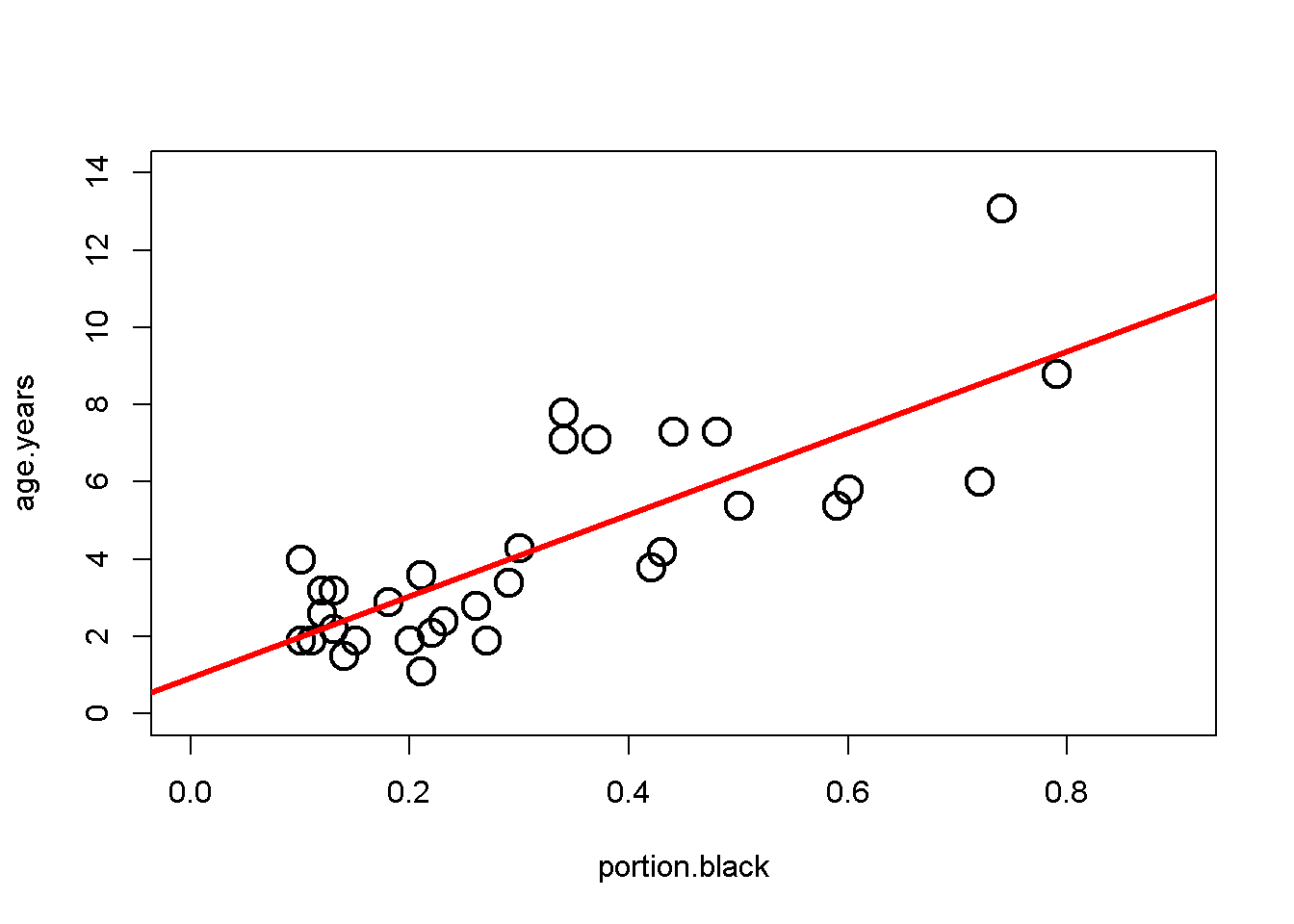#To Do: add equations for line

Plot lion data seperated by population

i.Serengeti <- which(lions$population == "Serengeti") i.Ngorogoro <- which(lions$population == "Ngorogoro")

#plot Serengeti
plot(age.years ~ portion.black,
data = lions,
subset = i.Serengeti,
cex = 2, lwd = 2,col = 2,
xlim = c(0.0, 0.9),
ylim = c(0,14))

#plot Serengeti
points(age.years ~ portion.black,
data = lions,
subset = i.Ngorogoro,
cex = 2, lwd = 2,
col = 3,pch = 2,
xlim = c(0.0, 0.9),
ylim = c(0,14))

legend("topleft",
legend = c("Serengeti","Ngorogoro"),
col = c(2,3),pch = c(1,2),cex = 1.2,
)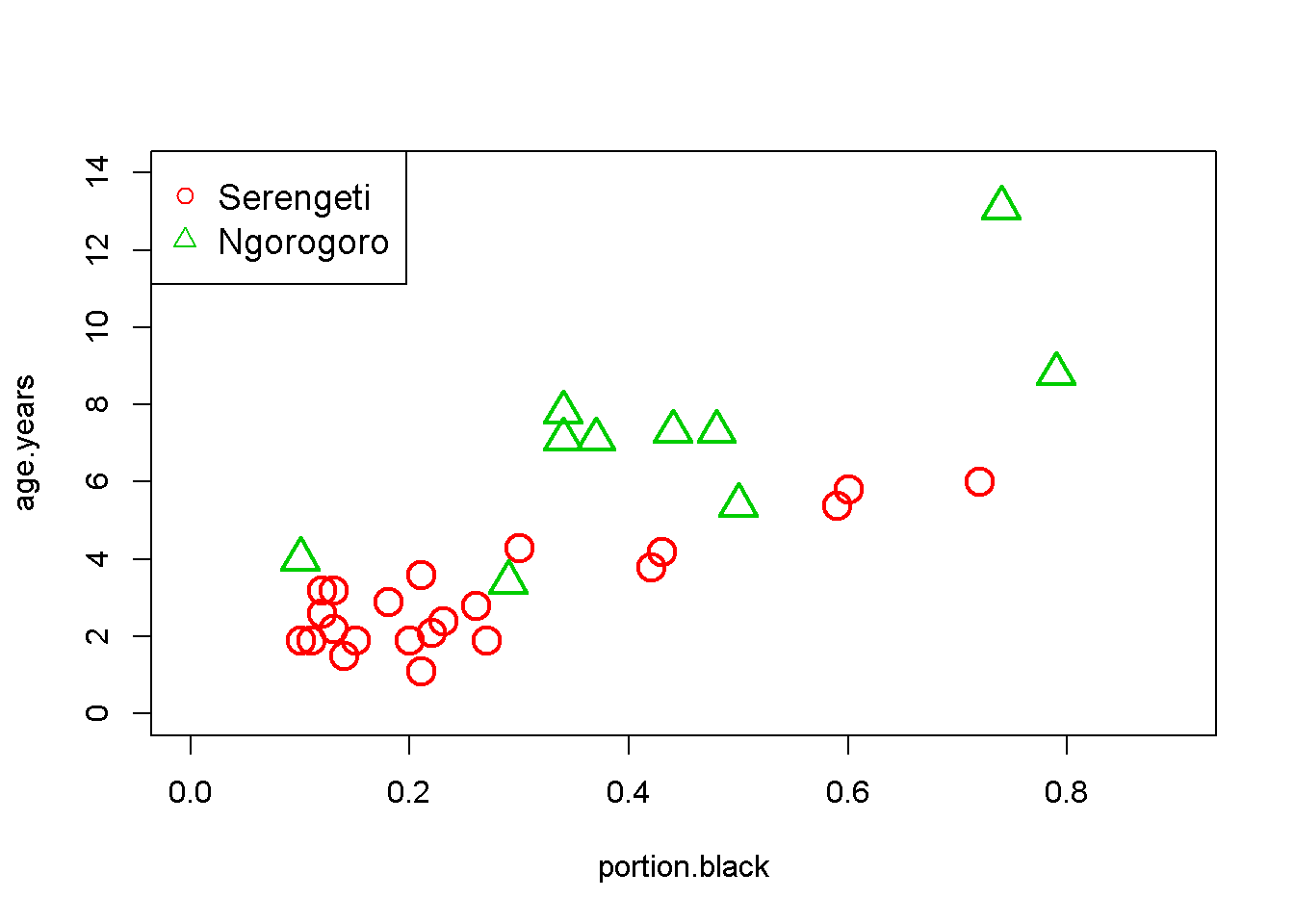What model best describes the data: 1 line or 2?

Hypothesis 0: there is no relationship

The null hypothesis Ho is that there is no relationship between how black the nose is and age. We can see that there is a relationship and will skip this Ho.

Hypothesis 1: the populations are the same

The data can be modeled with a single regression line. Any difference between the populations due to random noise.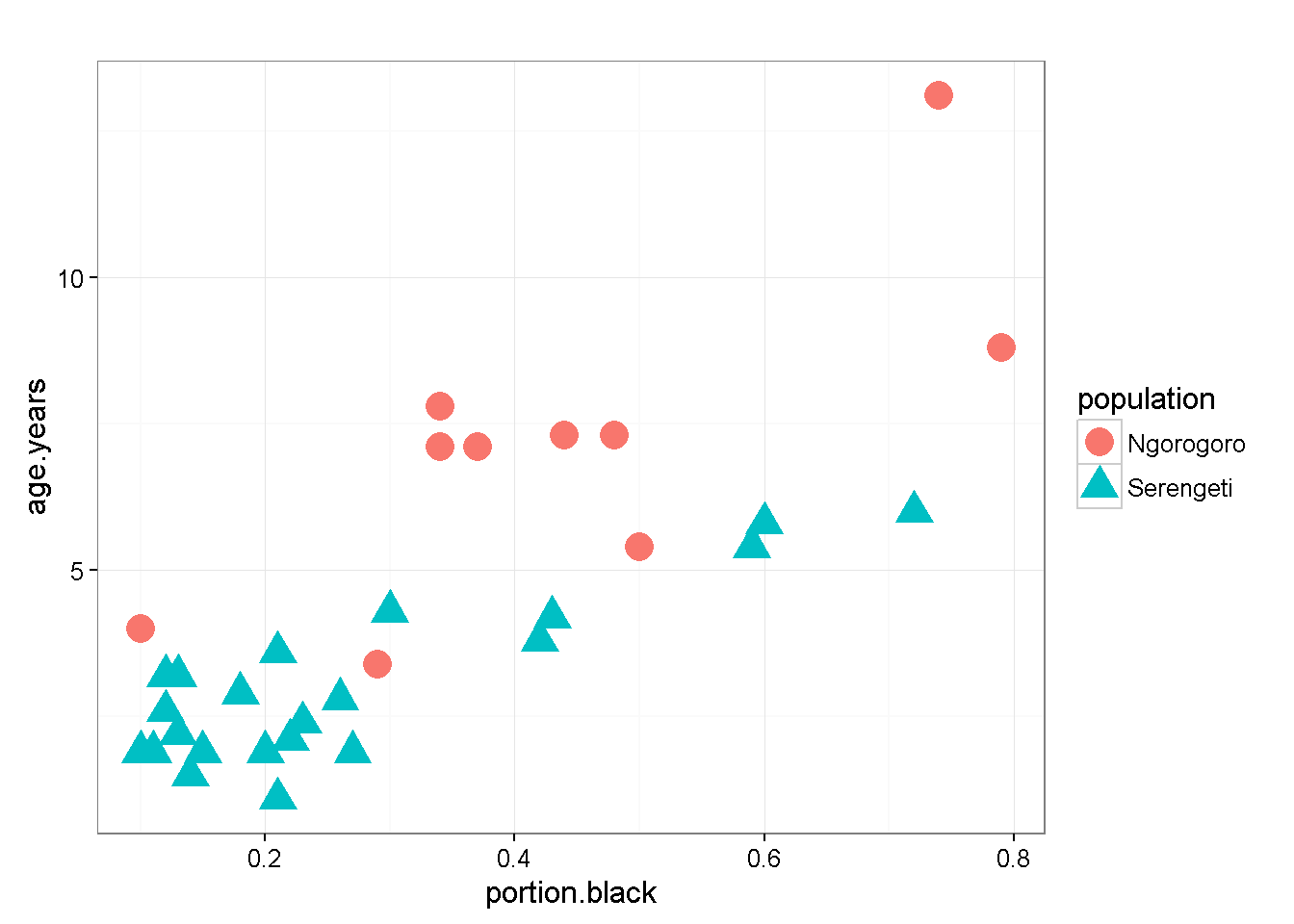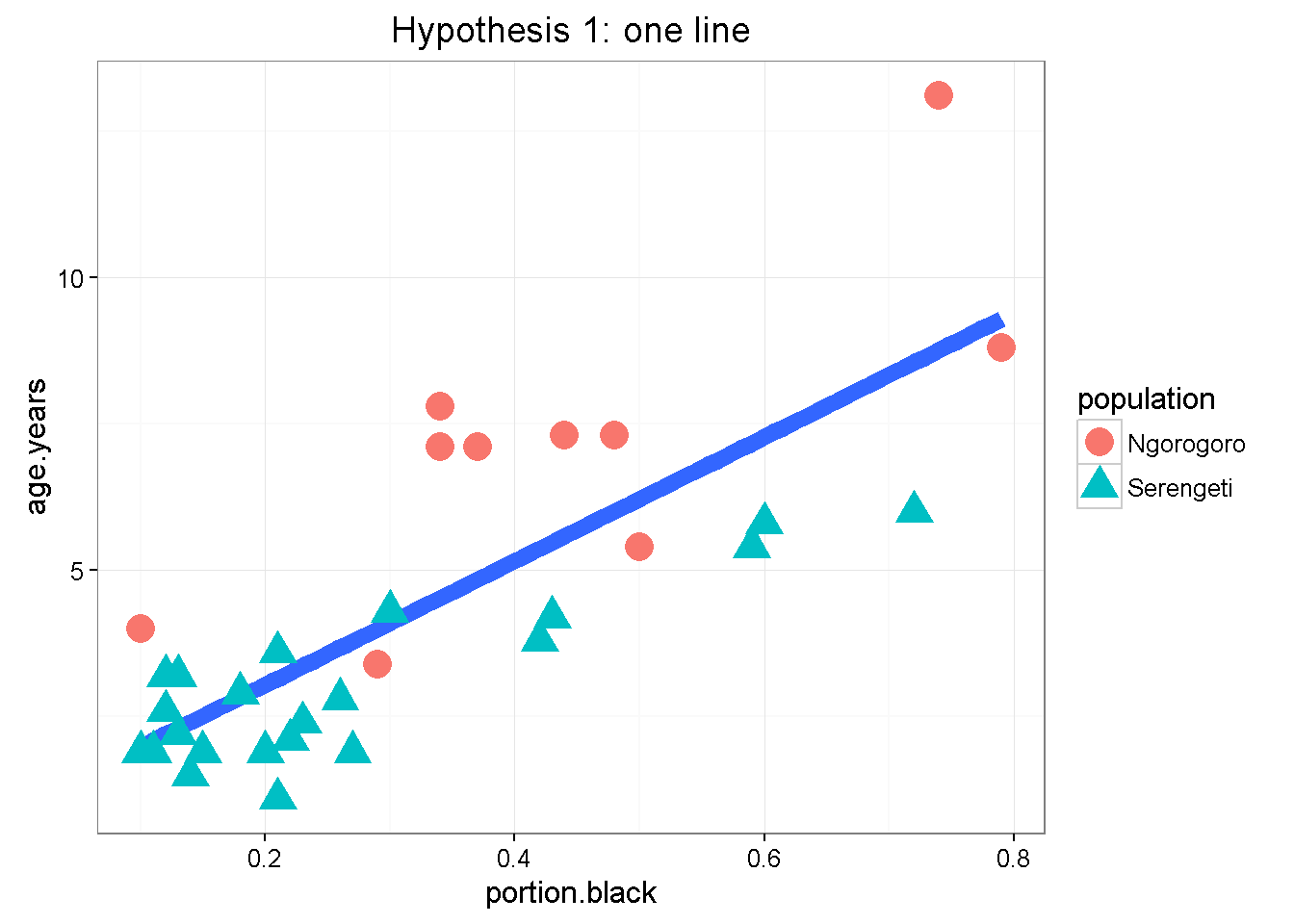Hypothesis 2: the populations are different

The populations have different biological/ecological processes going on that make the relationship between age and nose blackness depend on the population We say there is an INTERACTION between population and portion.black stated another way: The relationship between nose blackness and age is different between the two populations Therefore, the slope of the lines are different *(This include the possibility that there is no relationship in one population (slope = 0) and is a relationship in the other (slope >0) )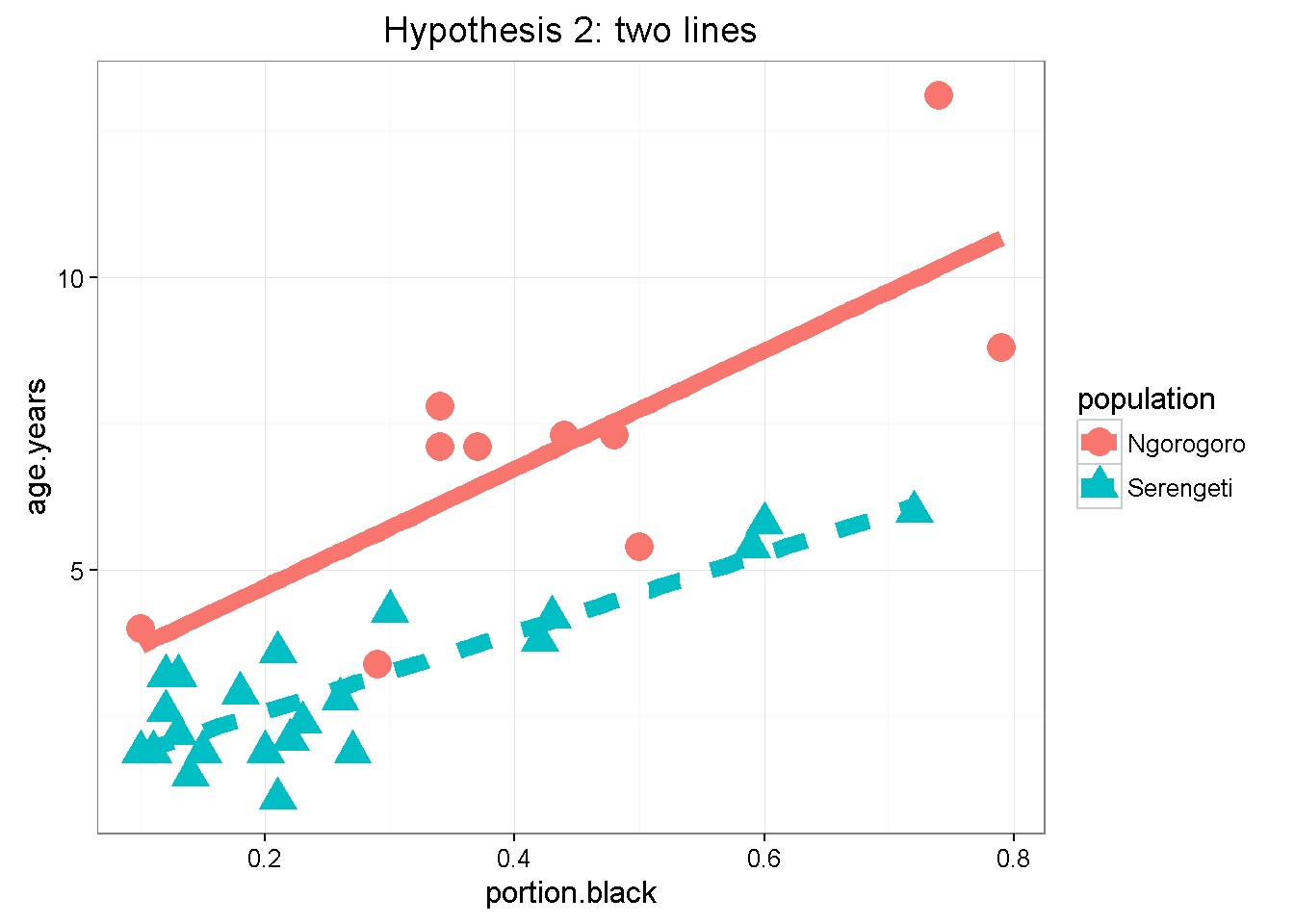Run multiple regression model

*We indicate in interaction in R using the multiple symbol “*"

Look at multiple regression output

summary(m.by.pop)
##
## Call:
## lm(formula = age.years ~ portion.black * population, data = lions)
##
## Residuals:
##     Min      1Q  Median      3Q     Max
## -2.3453 -0.4460  0.0258  0.5827  2.9336
##
## Coefficients:
##                                   Estimate Std. Error t value Pr(>|t|)
## (Intercept)                         2.7015     0.9032   2.991  0.00574 **
## portion.black                      10.0877     1.8801   5.365 1.02e-05 ***
## populationSerengeti                -1.4667     1.0106  -1.451  0.15781
## portion.black:populationSerengeti  -3.3352     2.3630  -1.411  0.16914
## ---
## Signif. codes:  0 '***' 0.001 '**' 0.01 '*' 0.05 '.' 0.1 ' ' 1
##
## Residual standard error: 1.16 on 28 degrees of freedom
## Multiple R-squared:  0.8303, Adjusted R-squared:  0.8122
## F-statistic: 45.68 on 3 and 28 DF,  p-value: 6.508e-11

Test models

Compare the model w/1 line vs. the model w/ 2 lines

anova(m.pooled, # data combined (aka "pooled")
m.by.pop) # data seperated by population
## Analysis of Variance Table
##
## Model 1: age.years ~ portion.black
## Model 2: age.years ~ portion.black * population
##   Res.Df    RSS Df Sum of Sq      F    Pr(>F)
## 1     30 82.712
## 2     28 37.679  2    45.034 16.733 1.657e-05 ***
## ---
## Signif. codes:  0 '***' 0.001 '**' 0.01 '*' 0.05 '.' 0.1 ' ' 1

MOre stuff

#no relationship
m.null <- lm(age.years ~ 1,data = lions)

#relationship is the same for both populations
m.pooled <- lm(age.years ~ portion.black,data = lions)

#slope is the same but intercepts are different
m.by.pop.add <- lm(age.years ~ portion.black + population,data = lions)

#slopes AND intercepts are different
m.by.pop.intnx <- lm(age.years ~ portion.black*population,data = lions)
#relationship is the same for both populations
summary(m.pooled)
##
## Call:
## lm(formula = age.years ~ portion.black, data = lions)
##
## Residuals:
##     Min      1Q  Median      3Q     Max
## -2.5457 -1.1457 -0.3384  0.9245  4.3426
##
## Coefficients:
##               Estimate Std. Error t value Pr(>|t|)
## (Intercept)     0.9262     0.5591   1.657    0.108
## portion.black  10.5827     1.4884   7.110 6.59e-08 ***
## ---
## Signif. codes:  0 '***' 0.001 '**' 0.01 '*' 0.05 '.' 0.1 ' ' 1
##
## Residual standard error: 1.66 on 30 degrees of freedom
## Multiple R-squared:  0.6276, Adjusted R-squared:  0.6152
## F-statistic: 50.55 on 1 and 30 DF,  p-value: 6.59e-08
#slope is the same but intercepts are different
##
## Call:
## lm(formula = age.years ~ portion.black + population, data = lions)
##
## Residuals:
##     Min      1Q  Median      3Q     Max
## -2.5415 -0.5361 -0.1484  0.5990  3.5691
##
## Coefficients:
##                     Estimate Std. Error t value Pr(>|t|)
## (Intercept)           3.6283     0.6306   5.753 3.14e-06 ***
## portion.black         7.9764     1.1582   6.887 1.45e-07 ***
## populationSerengeti  -2.7185     0.4928  -5.517 6.04e-06 ***
## ---
## Signif. codes:  0 '***' 0.001 '**' 0.01 '*' 0.05 '.' 0.1 ' ' 1
##
## Residual standard error: 1.18 on 29 degrees of freedom
## Multiple R-squared:  0.8183, Adjusted R-squared:  0.8057
## F-statistic: 65.29 on 2 and 29 DF,  p-value: 1.827e-11
#slopes AND intercepts are different
summary(m.by.pop.intnx)
##
## Call:
## lm(formula = age.years ~ portion.black * population, data = lions)
##
## Residuals:
##     Min      1Q  Median      3Q     Max
## -2.3453 -0.4460  0.0258  0.5827  2.9336
##
## Coefficients:
##                                   Estimate Std. Error t value Pr(>|t|)
## (Intercept)                         2.7015     0.9032   2.991  0.00574 **
## portion.black                      10.0877     1.8801   5.365 1.02e-05 ***
## populationSerengeti                -1.4667     1.0106  -1.451  0.15781
## portion.black:populationSerengeti  -3.3352     2.3630  -1.411  0.16914
## ---
## Signif. codes:  0 '***' 0.001 '**' 0.01 '*' 0.05 '.' 0.1 ' ' 1
##
## Residual standard error: 1.16 on 28 degrees of freedom
## Multiple R-squared:  0.8303, Adjusted R-squared:  0.8122
## F-statistic: 45.68 on 3 and 28 DF,  p-value: 6.508e-11